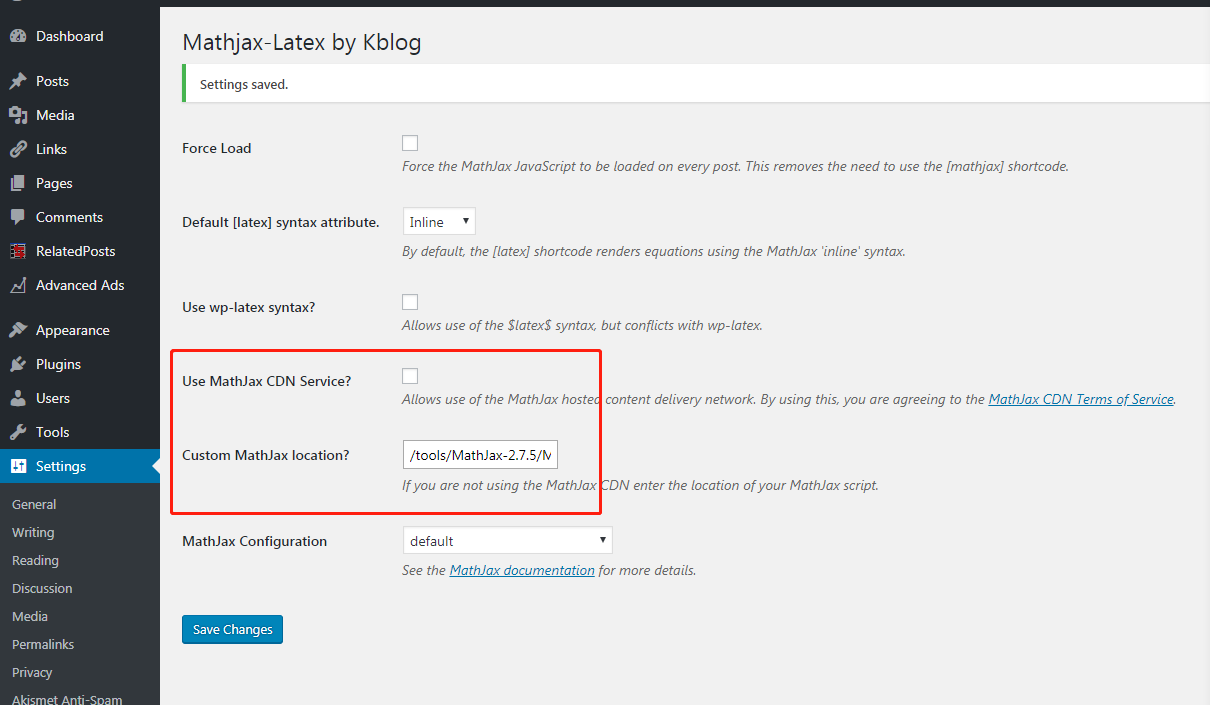## 伯努利(Binomial)分布和伯努利试验的区别，负伯努利分布及几何分布,超几何分布

#### 伯努利分布和伯努利试验的区别

$$b(x;n,p) = {n \choose x} p^k (1-p)^{ n-k}$$

### 什么是负伯努利分布(Negative Binomial Distribution)

$$b^*(x;k, p) = {{x-1} \choose {k-1}}p^kq^x-k$$

### 伯努利分布，负伯努利分布的命名

• 为什么叫伯努利分布
因为伯努利展开式$$(q+p)^n$$的n+1个项对应伯努利分布取各个值时的情况。
• 为什么叫负伯努利分布
因为展开式$$p^k(1-p)^(-k)$$中的每个项对应$$b^*(x;k,p)$$取x=k, k+1, k+2, …时的情况。

## 安装MathJax-LaTeX插件，让wordpress显示数学公式

MathJax-LaTex插件依赖MathJax这个javascript开源工程。原因貌似是MathJax默认用的CDN在大陆被墙了，还是因为浏览器的同源策略被浏览器禁了??我懒得重现排查真正原因了。总之，我用MathJax-LaTex默认配置没有成功，于是我自己手动下载并安装到自己的服务器，运行OK。

1. 首先到 https://github.com/mathjax/MathJax 下载源代码。熟悉git的同学可以用git下载，亦可用右边的 Clone or Dowload 按钮下载zip包。
2. 把zip包上传到自己的服务器，找一个目录解压缩。
3. 打开MathJax-LaTex的配置web页面，如下图如示，去掉Use MathJax CDN Service 的勾，不要用MathJax自己的CDN；然后，在Custom MathJax location选项中填上自己的路径。假如刚才zip包MathJax解压缩到的目录是/tools，那么就填上 /tools/MathJax-2.7.5/MathJax.js4. 然后创建一篇新博文，现在可以录入数学公式啦。装逼必备！比如下面的伯努利分布的公式：

$$b(x;n,p) = {n \choose x} p^k (1-p)^{ n-x}$$